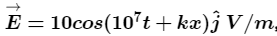# Problem: The electric field of an electromagnetic wave in free space is given bywhere t and x are in seconds and meters respectively. It can be inferred that(i) the wavelength λ is 188.4 m.(ii) the wave number k is 0.33 rad/m.(iii) the wave amplitude is 10 V/m.(iv) the wave is propagating along +x direction.Which one of the following pairs of statements is incorrect?A. i and iiB. ii and iiiC. i and iiiD. iii and iv

###### FREE Expert Solution

The standard equation for electromagnetic wave:

$\overline{){\mathbf{E}}{\mathbf{=}}{{\mathbf{E}}}_{{\mathbf{0}}}{\mathbf{c}}{\mathbf{o}}{\mathbf{s}}{\mathbf{\left(}}{\mathbf{\omega }}{\mathbf{t}}{\mathbf{+}}{\mathbf{k}}{\mathbf{x}}{\mathbf{\right)}}}$

With the given equation:

E0 = 10 V/m

ω = 107

Therefore, c = vλ = ωλ/2π

81% (61 ratings)###### Problem Details

The electric field of an electromagnetic wave in free space is given bywhere t and x are in seconds and meters respectively. It can be inferred that

(i) the wavelength λ is 188.4 m.
(ii) the wave number k is 0.33 rad/m.
(iii) the wave amplitude is 10 V/m.
(iv) the wave is propagating along +x direction.

Which one of the following pairs of statements is incorrect?

A. i and ii

B. ii and iii

C. i and iii

D. iii and iv